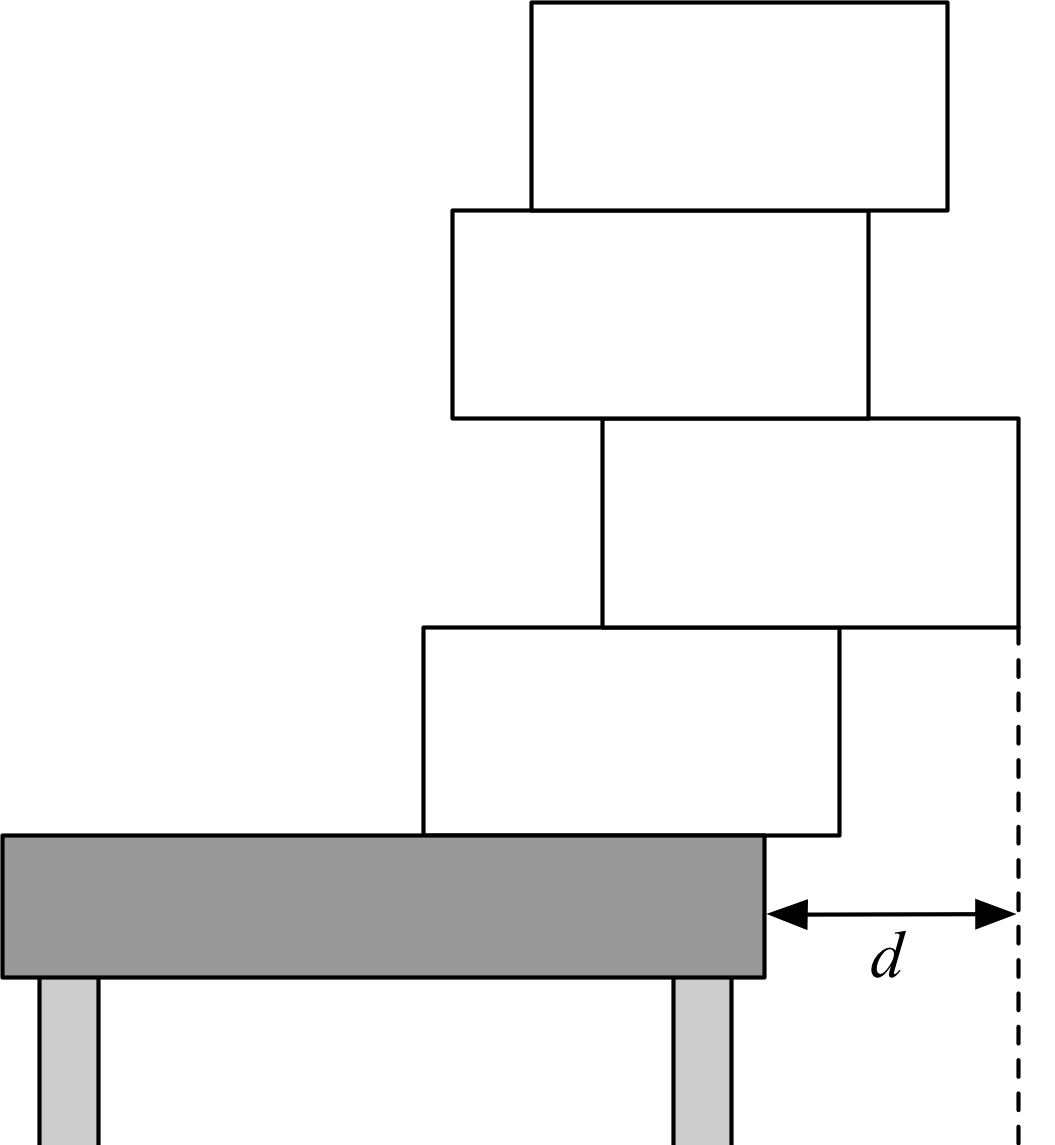# 3511. 小学物理期末考试### 输入格式

$1$ 行包含 $1$ 正整数 $n$。($1 \leq n \leq 500~000$)

$2$ 行包含 $n$ 个正整数，第 $i$ 个表示 $M_i$。($1 \leq M_i \leq 500~000$)

### 样例

Input
2
1 1

Output
1.500000


3 人解决，20 人已尝试。

4 份提交通过，共有 102 份提交。

9.5 EMB 奖励。